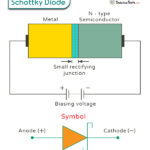Home / Physics / Newton’s Third Law

# Newton’s Third Law

## What is Newton’s Third Law

Newton’s third law states that “If one object exerts a force on another object, then the other object exerts an equal and opposite force on the first object”.

According to Newton’s third law, whenever two objects interact, they apply a force on each other. In other words, forces must always occur in pairs. These forces are known as action and reaction, and hence, another name for Newton’s third law is action-reaction law. Therefore, for every action, there is an equal and opposite reaction. Newton’s third law is applicable to bodies in rest or motion.

An example of Newton’s third law is a book lying on a table, as shown in the image below. The book applies a force on the table, which is the book’s weight. In order to prevent deformation, the table exerts an equal and opposite force on the book. This force is the normal force.

## Newton’s Third Law Examples

1. Rocket Launch

A rocket’s thrust produces a large amount of force during launching. This force is responsible for lifting the rocket into space. Here, the thrust is the action, and the lift is the reaction. Both forces are equal and opposite.

2. Birds Flying

Birds can fly because of wings. The wings push the air downwards. The air imparts an upward force on the wings allowing the birds to lift off the ground.

3. Car Moving

The wheels of a moving car push against the road with force. In turn, the road applies a forward friction force on the wheel that prevents the car from slipping. The two forces balance each other so that the car can ride smoothly without skidding. The wheel and axle is an example of simple device that obeys Newton’s third law.

4. Swimming

An example of Newton’s third law in sport is swimming. A swimmer pushes the water backward with his arms. The water reacts by applying a force in the forward direction enabling the swimmer to move ahead.

5. Earth and Moon

The Earth exerts a gravitational force on the Moon. The Moon exerts an equal and opposite force on Earth. The two forces are equal and opposite, thus enabling the Moon to revolve around the Earth’s orbit.

6. Two Magnets

When the like poles of two magnets are brought close to one another, they repel. The repulsive force exerted by the first magnet on the second is equal and opposite to the repulsive force exerted by the second magnet on the first.

7. Rowing a Boat

While rowing a boat, a person pushes the water backward with the oars. It is the action. The water applies an equal and opposite force on the boat that pushes the boat forward. It is the reaction.

## Newton’s Third Law Equation

Suppose an object A exerts a force FAB on object B, and the latter exerts a force FBA on the former. Then, Newton’s third law can be written as,

FAB = – FBA

In other words,

Action = – ReactionSchottky DiodeBipolar Junction TransistorHalf-Wave RectifierFull Wave Rectifier